# Calculus - Calculus MCQ

31:

The function f(x) = x3 - 6x2 + 3x + 25 has

 A. a maxima at x = 1 and a minima at x = 3 B. a maxima at x = 3 and a minima at x = 1 C. no maxima, but a _minima at x = 1 D. a maxima at x = 1, but no minima Answer Report Discuss Option: A Explanation : f ' (x) = 3(x2 - 4x + 3), f '' (x) = 6(x) - 12 At     x = 1, f "(1) At     x = 3, f " (3) = + ve  Click on Discuss to view users comments. Write your comments here:
32:

Integration ∫ log x dx has the value

 A. x log x - 1 B. log x - x C. x(log x -1) D. none of these Answer Report Discuss Option: C Explanation :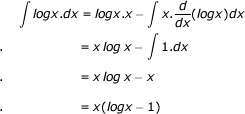Click on Discuss to view users comments. Write your comments here:
33:

The integral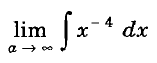A. diverges B. converges to 1/3 C. converges to  - 1 / a3 D. converges to 0 Answer Report Discuss Option: B Explanation :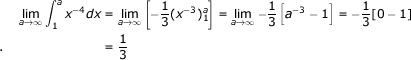Click on Discuss to view users comments. Write your comments here:
34:

If f(x), φ(x) and ψ (x) have derivatives when a ≤ x ≤ b, then E a value of c of x lying between a and b such that

 A.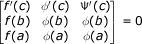B.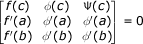C.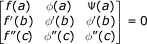D. none of these Answer Report Discuss Option: C Explanation : Click on Discuss to view users comments. Write your comments here:
35:

The limit of the function f(x) = [ 1 - a4 / x4 ] as x ---> ∞ is given by

 A. 1 B. exp[ -a4 ] C. ∞ D. zero Answer Report Discuss Option: A Explanation :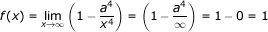Click on Discuss to view users comments. Write your comments here: